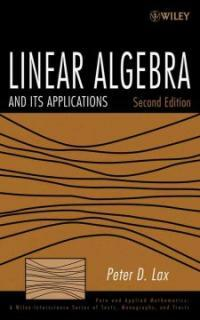> Detail View

# Detail View## Linear algebra and its applications 2nd ed (Loan 16 times)

Material type
단행본
Personal Author
Lax, Peter D. Lax, Peter D.
Title Statement
Linear algebra and its applications / Peter D. Lax.
판사항
2nd ed.
Publication, Distribution, etc
Hoboken, N.J. :   John Wiley,   c2008.
Physical Medium
xv, 376 p. : ill. ; 25 cm.
Series Statement
Pure and applied mathematics. A Wiley-Interscience of texts, monographs and tracts
ISBN
0471751561 9780471751564 (cloth)
General Note
Previous ed.: Linear algebra. New York : Wiley, c1997.
Content Notes
Fundamentals -- Duality -- Linear mappings -- Matrices -- Determinant and trace -- Spectral theory -- Euclidean structure -- Spectral theory of self-adjoint mappings -- Of a Euclidean space into itself -- Calculus of vector and matrix-valued functions -- Matrix inequalities -- Kinematics and dynamics -- Convexity -- The duality theorem -- Normed linear spaces -- Linear mappings between normed linear spaces -- Positive matrices -- How to solve systems of linear equations -- How to calculate the eigenvalues of self-adjoint matrices -- Solutions of selected exercises.
Bibliography, Etc. Note
Includes bibliographical references and index.
Algebras, Linear.
 000 01751camuu2200337 a 4500 001 000045419529 005 20110303185529 008 070601s2008 njua b 001 0 eng 010 ▼a 2007023226 020 ▼a 0471751561 020 ▼a 9780471751564 (cloth) 035 ▼a (KERIS)REF000013120430 040 ▼a DLC ▼c DLC ▼d DLC ▼d 211009 050 0 0 ▼a QA184.2 ▼b .L38 2008 082 0 0 ▼a 512/.5 ▼2 22 084 ▼a 512.5 ▼2 DDCK 090 ▼a 512.5 ▼b L4252L2 100 1 ▼a Lax, Peter D. 245 1 0 ▼a Linear algebra and its applications / ▼c Peter D. Lax. 250 ▼a 2nd ed. 260 ▼a Hoboken, N.J. : ▼b John Wiley, ▼c c2008. 300 ▼a xv, 376 p. : ▼b ill. ; ▼c 25 cm. 490 1 ▼a Pure and applied mathematics. A Wiley-Interscience of texts, monographs and tracts 500 ▼a Previous ed.: Linear algebra. New York : Wiley, c1997. 504 ▼a Includes bibliographical references and index. 505 0 ▼a Fundamentals -- Duality -- Linear mappings -- Matrices -- Determinant and trace -- Spectral theory -- Euclidean structure -- Spectral theory of self-adjoint mappings -- Of a Euclidean space into itself -- Calculus of vector and matrix-valued functions -- Matrix inequalities -- Kinematics and dynamics -- Convexity -- The duality theorem -- Normed linear spaces -- Linear mappings between normed linear spaces -- Positive matrices -- How to solve systems of linear equations -- How to calculate the eigenvalues of self-adjoint matrices -- Solutions of selected exercises. 650 0 ▼a Algebras, Linear. 700 1 ▼a Lax, Peter D. ▼t Linear algebra. 830 0 ▼a Pure and applied mathematics. ▼p A Wiley-Interscience of texts, monographs and tracts. 945 ▼a KINS

### Holdings Information

No. Location Call Number Accession No. Availability Due Date Make a Reservation Service
No. 1 Location Call Number 512.5 L4252L2 Accession No. 121164303 Availability Available Due Date Make a Reservation Service
No. 2 Location Call Number 512.5 L4252L2 Accession No. 121206509 Availability Available Due Date Make a Reservation Service

### Contents information

`Preface.  Preface to the First Edition. 1. Fundamentals. 2. Duality. 3. Linear Mappings. 4. Matrices. 5. Determinant and Trace. 6. Spectral Theory. 7. Euclidean Structure. 8. Spectral Theory of Self-Adjoint Mappings. 9. Calculus of Vector- and Matrix-Valued Functions. 10. Matrix Inequalities. 11. Kinematics and Dynamics. 12. Convexity. 13. The Duality Theorem. 14. Normed Linear Spaces. 15. Linear Mappings Between Normed Linear Spaces. 16. Positive Matrices. 17. How to Solve Systems of Linear Equations. 18. How to Calculate the Eigenvalues of Self-Adjoint Matrices. 19. Solutions. Bibliography. Appendix 1. Special Determinants. Appendix 2. The Pfaffian. Appendix 3. Symplectic Matrices. Appendix 4. Tensor Product. Appendix 5. Lattices. Appendix 6. Fast Matrix Multiplication. Appendix 7. Gershgorin's Theorem. Appendix 8. The Multiplicity of Eigenvalues. Appendix 9. The Fast Fourier Transform. Appendix 10. The Spectral Radius. Appendix 11. The Lorentz Group. Appendix 12. Compactness of the Unit Ball. Appendix 13. A Characterization of Commutators. Appendix 14. Liapunov's Theorem. Appendix 15. The Jordan Canonical Form. Appendix 16. Numerical Range. Index.`

Information Provided By: :김대수 (2022)

민만식 (2022)